# MA.912.F.2.1Export Print
Identify the effect on the graph or table of a given function after replacing f(x) by f(x)+k,kf(x), f(kx) and f(x+k) for specific values of k.

### Clarifications

Clarification 1: Within the Algebra 1 course, functions are limited to linear, quadratic and absolute value.

Clarification 2: Instruction focuses on including positive and negative values for k.

General Information
Subject Area: Mathematics (B.E.S.T.)
Strand: Functions
Status: State Board Approved

## Benchmark Instructional Guide

### Terms from the K-12 Glossary

• Transformation
• Translation

### Vertical Alignment

Previous Benchmarks

Next Benchmarks

### Purpose and Instructional Strategies

In grade 8, students performed single transformations on two-dimensional figures. In Algebra I, students identify the effects of single transformations on linear, quadratic and absolute value functions. In Geometry, students will perform multiple transformations on two-dimensional figures. In later courses, student will work with transformations of many types of functions.
• In this benchmark, students will examine the impact of transformations on linear, quadratic, and absolute value functions. Instruction includes the use of graphing software to ensure adequate time for students to examine multiple transformations on the graphs of functions.
• Have students use graphing technology to explore different parent functions.
• In each graph, toggle on/off the graphs for $f$($x$) + $k$, $k$$f$($x$), $f$($k$$x$) and $f$($x$ + $x$) to examine their impacts on the function. Use the slider to change the value of $k$ (be sure to examine the impacts when $k$ is positive and negative).
• As students explore, prompt discussion (MTR.4.1) among them about the patterns they see as they adjust the slider (MTR.5.1).
• For $f$($x$) + $k$, students should discover that $k$ is being added to the output of the function (equivalent to the $y$-value) and will therefore result in a vertical translation of the function by $k$ units.
• Ask students to describe what values of $k$ cause the graph to shift up. Which values cause it to shift down?
• For $k$$f$($x$), students should discover that $k$ is being multiplied by the output of the function (equivalent to the $y$-value) and will therefore result in a vertical dilation (stretch/compression) of the function by a factor of $k$
• Ask students to describe what values of $k$ cause the graph to stretch up vertically. Which values cause it to compress? Which values for $k$ cause the graph to reflect over the $x$-axis? What is the significance of $k$ = −1?
• For $f$($x$ + $k$), students should discover that $k$ is being added to the input of the function and will therefore result in a horizontal translation of the function by −$k$ units.
• Ask students to describe what values of $k$ cause the graph to shift left. Which values cause it to shift right?
• For $f$($k$$x$), students should discover that $k$ is being multiplied by the input of the function and will therefore result in a horizontal dilation (stretch/compression) of the function by a factor of $k$
• Ask students to describe what values of $k$ cause the graph to stretch horizontally. Which values cause it to compress? Which values for $k$ cause the graph to reflect over the $y$-axis? What is the significance of $k$ = −1?
• After students have a good understanding of the impact of $f$($x$) + $k$, $k$$f$($x$), $f$($k$$x$) and $f$($x$ + $k$) on graphs of functions, connect that knowledge to tables of values for a function.
• For $f$($x$) + $k$, use graphing technology to display a graph of a quadratic function (like the one below) and set $k$ = 4. Guide students to form a table and discuss its connection to the vertical translation observed on the graph.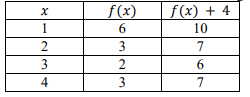• For $k$$f$($x$), use graphing technology to display a graph of a quadratic function (like the one below) and set $k$ = 0.5. Guide students to form a table and discuss its connection to the vertical compression observed on the graph.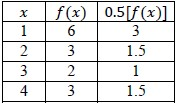• For $f$($x$ + $k$), use graphing technology to display a graph of a quadratic function (like the one below) and set $k$ = 2. Guide students to form a table and discuss its connection to the horizontal translation observed on the graph. This one may be tricky for students to understand initially. For the table shown, consider $x$ = 5. For $f$($x$), $f$(5) = 6. But for $g$($x$) = $f$($x$ + 2), $g$(5) = $f$(5 + 2) which is equivalent to 18, which is equivalent to shifting $f$(7) two units to the left on the graph. Bridge this conversation with a graph of the two functions to help them understand the connection.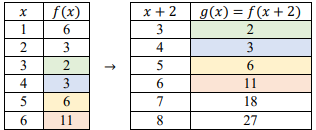• For $f$($k$$x$), use graphing technology to display a graph of a quadratic function (like the one below) and set $k$ = 3. Guide students to form a table and discuss its connection to the horizontal compression observed on the graph.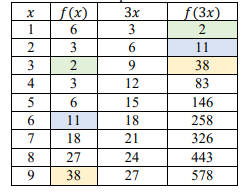### Common Misconceptions or Errors

• Similar to writing functions in vertex form, students may confuse effect of the sign of $k$ in $f$($x$ + $k$). Direct these students to examine a graph of the two functions to see that the horizontal shift is opposite of the sign of $k$
• Vertical stretch/compression can be hard for students to see on linear functions initially and they may interpret stretch/compression as rotation. Introduce the effects of $k$$f$($x$) and $f$($k$$x$) by using a quadratic or absolute value function first before analyzing the effect on a linear function.
• Students may think that a vertical and horizontal stretch from $k$$f$($x$) and $f$($k$$x$) look the same. For linear and quadratic functions, it can help to have a non-zero $y$-intercept to visualize the difference.

### Strategies to Support Tiered Instruction

• Instruction includes explaining to students that horizontal shifts are “inside” of the function. Additionally, the teacher provides instruction to ensure understanding that the movement of the function is opposite of the sign that effects the horizontal shift.
• For example, teacher can provide the identification of the type of transformation and its effects to the below function.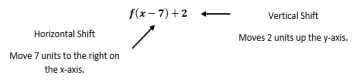• Teacher provides instruction that includes the use of a graph that displays stretch and compression scaling. Including a visual representation will allow students to categorize their thinking.
• For example, have students copy the graphs into their interactive notebooks. Give students an opportunity to identify changes in both types of transformations before giving students the transformations.
• Instruction includes providing a grid with a parent function and horizontal and vertical stretch on one grid, using different colors to distinguish both types of stretches (vertical and horizontal).
• Instruction includes having a non-zero $y$-intercept to visualize the difference between scaling in the horizontal direction, $f$($k$$x$), and scaling in the vertical direction, $k$$f$($x$).

• Part A. Given the function $f$($x$) = $x$2, determine the vertex, domain and range.
• Part B. If the function $f$($x$) is translated to the right 6 units, predict what may happen to the vertex, domain and range.
• Part C. How does the graph of the function $f$($x$) = $x$2 − 7, compare to the graph of the function in Part A?

### Instructional Items

Instructional Item 1
• How does the graph of $g$($x$) = $f$($x$) − 2 compare to the graph of $f$($x$) = |$x$ + 3|?
Instructional Item 2
• Describe the effect of the transformation $f$($x$) + 2 on the function table below.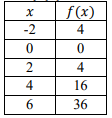*The strategies, tasks and items included in the B1G-M are examples and should not be considered comprehensive.

## Related Courses

This benchmark is part of these courses.
1200310: Algebra 1 (Specifically in versions: 2014 - 2015, 2015 - 2022, 2022 and beyond (current))
1200320: Algebra 1 Honors (Specifically in versions: 2014 - 2015, 2015 - 2022, 2022 and beyond (current))
1200370: Algebra 1-A (Specifically in versions: 2014 - 2015, 2015 - 2022, 2022 and beyond (current))
1200380: Algebra 1-B (Specifically in versions: 2014 - 2015, 2015 - 2022, 2022 and beyond (current))
1200400: Foundational Skills in Mathematics 9-12 (Specifically in versions: 2014 - 2015, 2015 - 2022, 2022 and beyond (current))
7912080: Access Algebra 1A (Specifically in versions: 2014 - 2015, 2015 - 2018, 2018 - 2019, 2019 - 2022, 2022 and beyond (current))
7912090: Access Algebra 1B (Specifically in versions: 2014 - 2015, 2015 - 2018, 2018 - 2019, 2019 - 2022, 2022 and beyond (current))
1200315: Algebra 1 for Credit Recovery (Specifically in versions: 2014 - 2015, 2015 - 2022, 2022 and beyond (current))
1200375: Algebra 1-A for Credit Recovery (Specifically in versions: 2014 - 2015, 2015 - 2022, 2022 and beyond (current))
1200385: Algebra 1-B for Credit Recovery (Specifically in versions: 2014 - 2015, 2015 - 2022, 2022 and beyond (current))
7912075: Access Algebra 1 (Specifically in versions: 2014 - 2015, 2015 - 2018, 2018 - 2019, 2019 - 2022, 2022 and beyond (current))
1200710: Mathematics for College Algebra (Specifically in versions: 2022 and beyond (current))

## Related Access Points

Alternate version of this benchmark for students with significant cognitive disabilities.
MA.912.F.2.AP.1:

Select the effect (up, down, left, or right) on the graph of a given function after replacing f(x) by f(x) + k and f(x + k) for specific values of k.

## Related Resources

Vetted resources educators can use to teach the concepts and skills in this benchmark.

## Formative Assessments

Students are given the graph of f(x) = x2 and are asked to compare the graphs of five other quadratic functions to the graph of f.

Type: Formative Assessment

Comparing Functions - Linear:

Students are asked to compare the graphs of four different linear functions to the graph of f(x) = x.

Type: Formative Assessment

## Lesson Plans

In this lesson, students will investigate the changes to the graph of a quadratic function when the function is modified in four different ways by inclusion of an additive or multiplicative constant. Students will work in groups to graph quadratic functions, prepare a display of their functions, and determine how the modification affects the graph of the quadratic function. Then, students participate in a gallery walk, where members of each group will share their findings with a small group of students. At the end, there is a class discussion to see if everyone had similar findings and to solidify the knowledge of translating quadratic functions.

Type: Lesson Plan

This is an introductory lesson to graphing quadratic equations. This lesson uses graphing technology to illustrate the differences between quadratic equations and linear equations. In addition, it allows students to identify important parts of the quadratic equation and how each piece changes the look of the graph.

Type: Lesson Plan

This is an introductory lesson to graphing quadratic equations. This lesson uses graphing technology to illustrate the differences between quadratic equations and linear equations. In addition, it allows students to identify important parts of the quadratic equation and how each piece changes the look of the graph.

Type: Lesson Plan

## Original Student Tutorials

Dilations...The Effect of k on a Graph:

Visualize the effect of using a value of k in both kf(x) or f(kx) when k is greater than zero in this interactive tutorial.

Type: Original Student Tutorial

Reflections...The Effect of k on a Graph:

Learn how reflections of a function are created and tied to the value of k in the mapping of f(x) to -1f(x) in this interactive tutorial.

Type: Original Student Tutorial

Translations...The Effect of k on the Graph:

Explore translations of functions on a graph that are caused by k in this interactive tutorial. GeoGebra and interactive practice items are used to investigate linear, quadratic, and exponential functions and their graphs, and the effect of a translation on a table of values.

Type: Original Student Tutorial

## MFAS Formative Assessments

Comparing Functions - Linear:

Students are asked to compare the graphs of four different linear functions to the graph of f(x) = x.

Students are given the graph of f(x) = x2 and are asked to compare the graphs of five other quadratic functions to the graph of f.

## Original Student Tutorials Mathematics - Grades 9-12

Dilations...The Effect of k on a Graph:

Visualize the effect of using a value of k in both kf(x) or f(kx) when k is greater than zero in this interactive tutorial.

Reflections...The Effect of k on a Graph:

Learn how reflections of a function are created and tied to the value of k in the mapping of f(x) to -1f(x) in this interactive tutorial.

Translations...The Effect of k on the Graph:

Explore translations of functions on a graph that are caused by k in this interactive tutorial. GeoGebra and interactive practice items are used to investigate linear, quadratic, and exponential functions and their graphs, and the effect of a translation on a table of values.

## Student Resources

Vetted resources students can use to learn the concepts and skills in this benchmark.

## Original Student Tutorials

Dilations...The Effect of k on a Graph:

Visualize the effect of using a value of k in both kf(x) or f(kx) when k is greater than zero in this interactive tutorial.

Type: Original Student Tutorial

Reflections...The Effect of k on a Graph:

Learn how reflections of a function are created and tied to the value of k in the mapping of f(x) to -1f(x) in this interactive tutorial.

Type: Original Student Tutorial

Translations...The Effect of k on the Graph:

Explore translations of functions on a graph that are caused by k in this interactive tutorial. GeoGebra and interactive practice items are used to investigate linear, quadratic, and exponential functions and their graphs, and the effect of a translation on a table of values.

Type: Original Student Tutorial

## Parent Resources

Vetted resources caregivers can use to help students learn the concepts and skills in this benchmark.